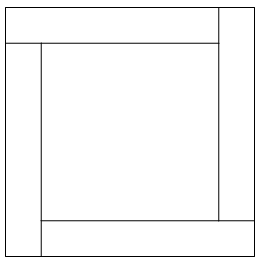# 1998 AHSME Problem 10

Geometry Level 1A large square is divided into a small square surrounded by four congruent rectangles as shown. The perimter of each of the congruent rectangles is 14. What is the area of the large square?

×